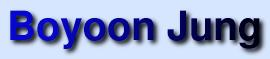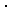Boyoon Jung's Library Manual

# bj::MDPf Class Reference [Markov Decision Process]

A Markov Decision Process Solver without storing matrices. More...

`#include <mdp.h>`

List of all members.

## Public Member Functions

MDPf (unsigned int nstates, unsigned int nactions)
A constructor.
~MDPf (void)
A destructor.
unsigned int * value_iteration (double discount=0.9, double epsilon=0.01, double *v0=0, int max_iter=-1)
Value iteration algorithm.
unsigned int * policy (void)
Return an optimal policy.
double * value_function (void)
Return a value function.
unsigned int numStates (void)
Return the number of states.
unsigned int numActions (void)
Return the number of actions.

## Protected Member Functions

virtual double T (unsigned int s, unsigned int a, unsigned int sp)=0
A transition probability function.
virtual double R (unsigned int s, unsigned int a)=0
A reward function.
void bellman_operation (unsigned int *p, double *v, double *vt_1, double discount)
Apply the bellman operation.
double span_semi_norm (double *vt, double *vt_1, unsigned int size)
Compute the span semi-norm.
void array_swap (double *&a0, double *&a1)
Swap two arrays.
void array_swap (unsigned int *&a0, unsigned int *&a1)
Swap two arrays.
double array_max (double *a, unsigned int size, unsigned int &index)
Find the maximum in an array.
double sub_matrix_min (unsigned int rows, unsigned int columns, unsigned int index)
Find the minimum sub-matrix.

## Protected Attributes

int num_states
State space size.
int num_actions
The number of actions.
unsigned int * P
An optimal policy.
double * V
A value function.
double * Vt_1
A value function in the previous step.
double * Q
A value-action function.

## Detailed Description

A Markov Decision Process Solver without storing matrices.

MDPf class provides a MDP (Markov Decision Process) solver. It provides only a value iteration algorithm. Since it does not store no transition probability matrix nor a reward matrix, it takes long time to compue an optimal policy. However, it requires only small amount of memory.

## Constructor & Destructor Documentation

 bj::MDPf::MDPf ( unsigned int nstates, unsigned int nactions )

A constructor.

Parameters:
 nstates The number of states. nactions The number of actions.

 bj::MDPf::~MDPf ( void )
 A destructor.

## Member Function Documentation

 double bj::MDPf::array_max ( double * a, unsigned int size, unsigned int & index ) ` [inline, protected]`
 Find the maximum in an array.

 void bj::MDPf::array_swap ( unsigned int *& a0, unsigned int *& a1 ) ` [inline, protected]`
 Swap two arrays.

 void bj::MDPf::array_swap ( double *& a0, double *& a1 ) ` [inline, protected]`
 Swap two arrays.

 void bj::MDPf::bellman_operation ( unsigned int * p, double * v, double * vt_1, double discount ) ` [inline, protected]`
 Apply the bellman operation.

 unsigned int bj::MDPf::numActions ( void ) ` [inline]`
 Return the number of actions.

 unsigned int bj::MDPf::numStates ( void ) ` [inline]`
 Return the number of states.

 unsigned int* bj::MDPf::policy ( void ) ` [inline]`
 Return an optimal policy.

 virtual double bj::MDPf::R ( unsigned int s, unsigned int a ) ` [protected, pure virtual]`
 A reward function. This function must be implemented in an inheriting class.

 double bj::MDPf::span_semi_norm ( double * vt, double * vt_1, unsigned int size ) ` [inline, protected]`
 Compute the span semi-norm.

 double bj::MDPf::sub_matrix_min ( unsigned int rows, unsigned int columns, unsigned int index ) ` [inline, protected]`
 Find the minimum sub-matrix.

 virtual double bj::MDPf::T ( unsigned int s, unsigned int a, unsigned int sp ) ` [protected, pure virtual]`
 A transition probability function. This function must be implemented in an inheriting class.

 double* bj::MDPf::value_function ( void ) ` [inline]`
 Return a value function.

 unsigned int* bj::MDPf::value_iteration ( double discount = `0.9`, double epsilon = `0.01`, double * v0 = `0`, int max_iter = `-1` )

Value iteration algorithm.

Parameters:
 discount A discount factor. [0 ~ 1] epsilon Search of an optimal policy. ]0 ~ 1] v0 An initial value function. max_iter Maximum number of iterations. If negative, a proper number of iterations is computed.

## Member Data Documentation

 int bj::MDPf::num_actions` [protected]`
 The number of actions.

 int bj::MDPf::num_states` [protected]`
 State space size.

 unsigned int* bj::MDPf::P` [protected]`
 An optimal policy.

 double* bj::MDPf::Q` [protected]`
 A value-action function.

 double* bj::MDPf::V` [protected]`
 A value function.

 double* bj::MDPf::Vt_1` [protected]`
 A value function in the previous step.

The documentation for this class was generated from the following file:
ResearchProjectsPublications

ToolsBjLib ManualPlayer/StagePyPlayerMicroStrain IMUGPS/DGPSParticle Filter

PersonalWelcomeCurriculum VitaeResumeContact

Outside LinksUSCUSC CS DeptCRESRobotics LabRESL